### Romer Model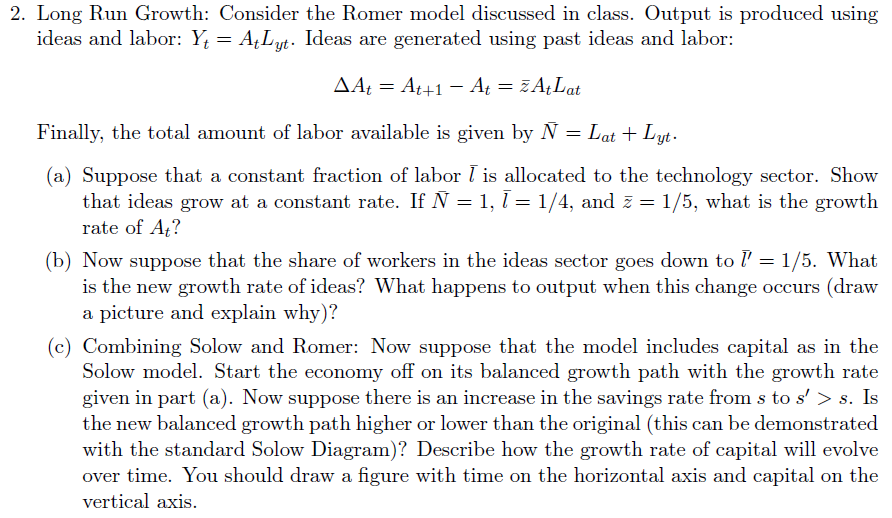Solutions Part A -Part C - Combining Solow and Romer(a) Be ready to show the work involved at pages 140-141 (Jones Macro Econ Crisis Update)Show that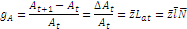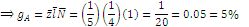The growth rate of A is five percent. (b) See page 146, Figure 6.4, but for this, we’re twisting clockwise (as opposed to Figure 6.4, which twists counter-clockwise). First, work through the algebra to get to equation 6.9 on page 141. You’ll conclude with the follow;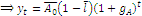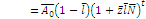With a decrease in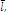, you can see there are two effects onFirstly, what is the new growth rate of A? (Growth Effect)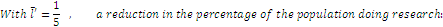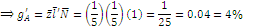This implies the clockwise twist down in growth lineSecondly (Level Effect), with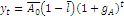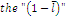implies that with a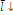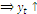immediately. An immediate bump to GDP-per-capita. Graphically, again, it’s figure 6.4, but twisted clockwise down – sort of the opposite of the figure in the book. To explain what is going on – read closely page 145-147. (Jones Macro Econ Crisis Update)The gist: there are two effects, growth effects, the change in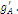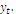~Be ready to explain the nuances of both, depending on the question Gorry delivers on the actual exam. Be ready to draw graphs that show the dynamics of experiments to the Romer model (like with figure 6.3 and 6.4)(c) see pages 158 – 163What is Growth Now?   From the Romer model, output per person is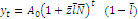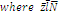is the growth rate of ideas.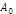is the initial stock of technology/ideas. And l is the portion of the population working on developing new ideas. The question asks to add capital, okay: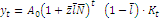The balanced growth path of this output-per-person would look just like the usual balanced growth path. Say, figure 6.2.Now, the question asked what happens if the savings rate were to jump up?Okay, from the Solow model (in long problem 1), we know that the steady state level of capital is:Thus if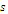increases, then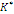takes a jump up. And that looks like the left panel of figure 5.5But remember that will Solow – you have no growth.is a steady state, constant level in the Solow model. In the Romer model things grow. They grow because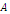,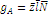. And this growth hasn't changed with a different savings rate. How Capital Evolves when the savings rate jumps up.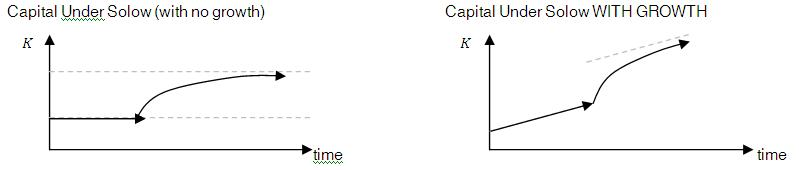The question is asking for the graph above to the right.  You can see capital is growing along there. Then the savings rate jumps up, and capital approaches the new steady state level of growth asymptotically.
Comments